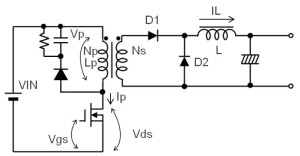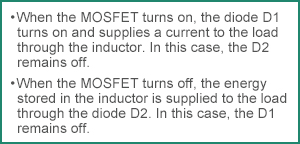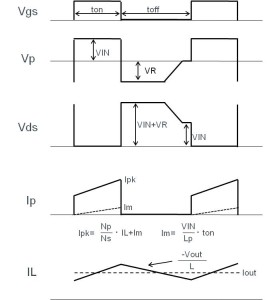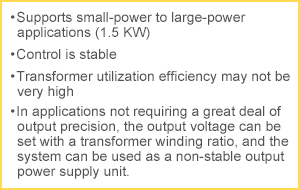# What is Forward System?

2015.09.03

･Though more complicated than the flyback system, on the secondary side the forward system operates according to the same underlying principle as in DC-DC conversion using diode rectification (asynchronous).

･As snubber circuits are also used extensively in power supply designs, we need a solid understanding of the underlying principles of those circuits.Figure 21. Forward system

Given a relatively simple configuration and easy to control, the forward system represents one of the more popular systems.

In terms of characteristics, the forward system is capable of producing larger-power output when compared with the flyback system, while requiring both an inductor and a flywheel diode (commutation diode: D2). In addition, similar to the flyback system, the forward system can be made into an insulated power supply unit through the use of a optocoupler on the feedback line from the output.Figure 22

The forward system operates as follows: When the MOSFET turns on, the diode D1 turns on and supplies a current to the load through the inductor. When the MOSFET turns off, the energy stored in the inductor is supplied in the form of a current to the load through the diode D2. Figure 23 shows waveforms for the key nodes.Figure 23. Forward system: Waveforms for the key nodes

Because the forward system excites the transformer only in one direction, while the transistor is off, the energy stored in the transformer must be released (reset). For this purpose, the forward system requires a reset (snubber) circuit (the RCD located on the primary side of the transformer in Figure 21). Generally a reset circuit is configured using a circuit containing a resistor, a capacitor, and a diode. However, because the energy ends up being lost, basically the utilization efficiency of the transformer may not be very high. In connection with the reset operation, a voltage as much as 1.5 to 2 times the DC input voltage is exerted on the switching transistor (in Figure 23, the VR in the Vp and Vds waveforms). This voltage varies with the snubber resistance and the capacitor. Recently, a system has come into use combining an active clamp that is capable of reducing losses and Vds by recovering the energy that needs to be reset.Figure 24

Furthermore, in the case of stepping down, the amount of current on the primary side is low, and the amount of energy trapped in the coils is not all that large. However, when the forward system is used for stepping up, the current on the primary side increases. Since the energy building up in the coils also varies as the square of the current, the energy lost in the reset circuit tends to be large. Consequently, although this circuit is used for stepping down operations, it is rarely used in stepping up applications.

In AC-DC conversions, the switching system is mainly used. Although it can also be used in the transformer system, similar to the flyback system, the use of the forward system is limited to applications that require adequate insulation.